Share

# Important Questions for SSC (Marathi Semi-English) 10th - Maharashtra State Board - Geometry

Subjects
Topics
Subjects
Popular subjects
Topics
Geometry
< prev 1 to 20 of 410 next >

In the following figure, in ΔPQR, seg RS is the bisector of ∠PRQ. If PS = 6, SQ = 8, PR = 15, find QR.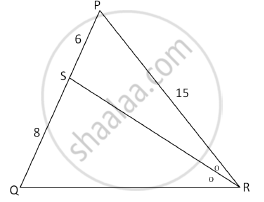Appears in 3 question papers
Chapter:  Similarity
Concept: Similarity of Triangles

If two circles with radii 8 cm and 3 cm, respectively, touch internally, then find the distance between their centers.

Appears in 3 question papers
Chapter:  Circle
Concept: Touching Circles

In Fig. 3, AP and BP are tangents to a circle with centre O, such that AP = 5 cm and ∠APB = 60°. Find the length of chord AB.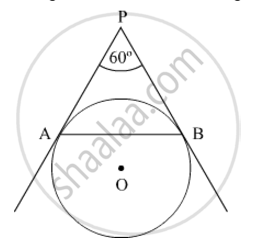Appears in 3 question papers
Chapter:  Mensuration
Concept: Areas of Sector and Segment of a Circle

A farmer connects a pipe of internal diameter 20 cm from a canal into a cylindrical tank which is 10 m in diameter and 2 m deep. If the water flows through the pipe at the rate of 4 km per hour, in how much time will the tank be filled completely?

Appears in 3 question papers
Chapter:  Mensuration
Concept: Surface Area of a Combination of Solids

In the following figure seg AB ⊥ seg BC, seg DC ⊥ seg BC. If AB = 2 and DC = 3, find (A(triangleABC))/(A(triangleDCB))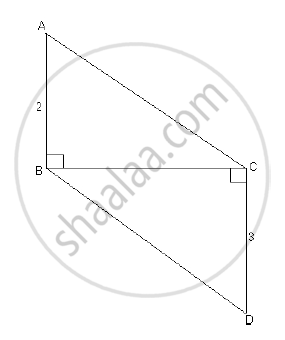Appears in 2 question papers
Chapter:  Similarity
Concept: Properties of Ratios of Areas of Two Triangles

Prove that the angle bisector of a triangle divides the side opposite to the angle in the ratio of the remaining sides.

Appears in 2 question papers
Chapter:  Similarity
Concept: Similarity of Triangles

In the following figure, seg BE ⊥ seg AB and seg BA ⊥ seg AD. If BE = 6 and $\text{AD} = 9 \text{ find} \frac{A\left( \Delta ABE \right)}{A\left( \Delta BAD \right)} \cdot$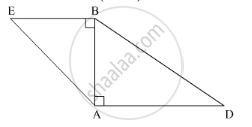Appears in 2 question papers
Chapter:  Similarity
Concept: Similarity of Triangles

In the given figure, AD is the bisector of the exterior ∠A of ∆ABC. Seg AD intersects the side BC produced in D. Prove that :

$\frac{BD}{CD} = \frac{AB}{AC}$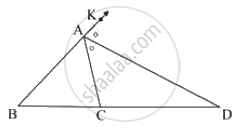Appears in 2 question papers
Chapter:  Similarity
Concept: Properties of Ratios of Areas of Two Triangles

Some question and their alternative answer are given. Select the correct alternative.

In a right angled triangle, if sum of the squares of the sides making right angle is 169 then what is the length of the hypotenuse?

Appears in 2 question papers
Chapter:  Pythagoras Theorem
Concept: Apollonius Theorem

Prove that “The lengths of the two tangent segments to a circle drawn from an external point are equal.”

Appears in 2 question papers
Chapter:  Circle
Concept: Number of Tangents from a Point on a Circle

If two circles with radii 5 cm and 3 cm respectively touch internally, find the distance between their centres.

Appears in 2 question papers
Chapter:  Circle
Concept: Touching Circles

Prove that ‘the opposite angles of a cyclic quadrilateral are supplementary’.

Appears in 2 question papers
Chapter:  Circle

Find the circumferences of a circle whose radius is 7 cm.

Appears in 2 question papers
Chapter:  Circle
Concept: Tangents and Its Properties

Prove that “The opposite angles of a cyclic quadrilateral are supplementary”.

Appears in 2 question papers
Chapter:  Circle
Concept: Cyclic Properties

Choose the correct alternative.

∠ACB is inscribed in arc ACB of a circle with centre O. If ∠ACB = 65°, find m(arc ACB).

Appears in 2 question papers
Chapter:  Circle
Concept: Inscribed Angle

A (5, 4), B (-3, -2) and C (1, -8) are the vertices of a triangle ABC. Find the equations of the median AD and line parallel to AC passing through the point B.

Appears in 2 question papers
Chapter:  Co-ordinate Geometry
Concept: General Equation of a Line

Find the slope and y-intercept of the line y = -2x + 3.

Appears in 2 question papers
Chapter:  Co-ordinate Geometry
Concept: Intercepts Made by a Line

Find the slope and y – intercept of the line y = 3x – 5.

Appears in 2 question papers
Chapter:  Co-ordinate Geometry
Concept: Intercepts Made by a Line

A (5, 4), B (–3,–2) and C (1,–8) are the vertices of a triangle ABC. Find the equation of median AD and line parallel to AB passing through point C.

Appears in 2 question papers
Chapter:  Co-ordinate Geometry
Concept: General Equation of a Line

ΔSHR ~ ΔSVU. In ΔSHR, SH = 4.5 cm, HR = 5.2 cm, SR = 5.8 cm and (SH)/(SV)=3/5. Construct ΔSVU.

Appears in 2 question papers
Chapter:  Geometric Constructions
Concept: Division of a Line Segment
< prev 1 to 20 of 410 next >
Important Questions for SSC (Marathi Semi-English) 10th Maharashtra State Board Geometry. You can further filter Important Questions by subjects and topics. Chapter wise important Questions for 10th Maharashtra State Board. it gets easy to find all 10th important questions with answers in a single place for students. Saving time and can then focus on their studies and practice. Important questions for 10th chapter wise with solutions

10th Geometry can be very challenging and complicated. But with the right ideas and support, you will be able to make it work. That’s why we have the 10th Geometry important questions with answers pdf ready for you. All you need is to acquire the PDF with all the content and then start preparing for the exam. It really helps and it can bring in front an amazing experience every time if you're tackling it at the highest possible level.